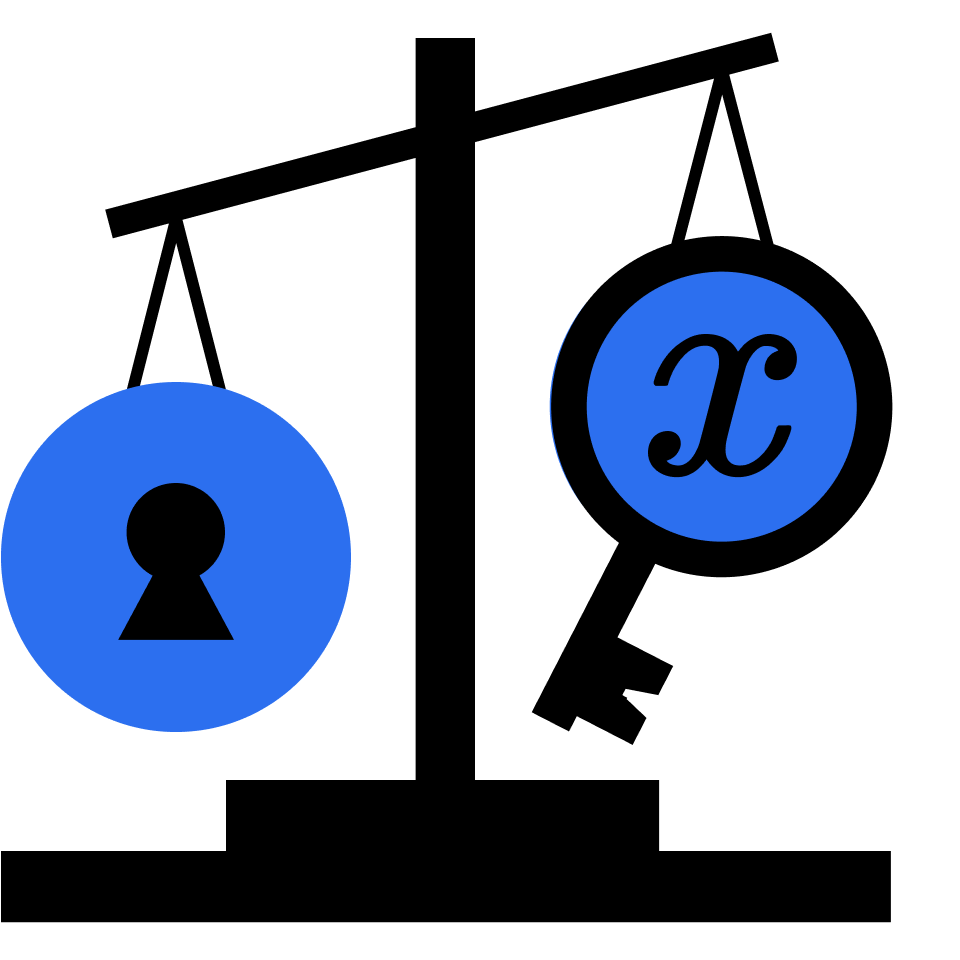## Solving Equations

Start your algebra journey here with an introduction to variables and equations.

Understanding Variables

Practice Understanding Variables

Understanding Expressions

Practice Understanding Expressions

Understanding Equations

Practice Understanding Equations

Grocery Shopping

Understanding Solutions

Practice Understanding Solutions

Working Backward

Practice Working Backward

Isolating Variables

Practice Isolating Variables

Isolating Expressions

Practice Isolating Expressions

Choosing a Credit Card

Writing Equations

Practice Writing Equations

More Operations on Signed Numbers

Practice More Operations on Signed Numbers

The Distributive Property

Practice The Distributive Property

Applying the Distributive Property

Practice Applying the Distributive Property

Combining Like Terms

Practice Combining Like Terms

Simplifying Equations

Practice Simplifying Equations

Representing Inequalities

Practice Representing Inequalities

Solving Inequalities

Practice Solving Inequalities

Handling Negatives

Practice Handling Negatives

Solving More Inequalities

Practice Solving More Inequalities

### Course description

Use diagrams and words to explore the very start of algebra, with puzzles and intuition guiding the way. By the end of the course, you'll be able to simplify expressions and solve equations using methods that impart deep understanding rather than just procedures. You'll sharpen your problem solving strategies and explore what's really useful about algebra in the first place.

### Topics covered

• Bar Models
• Distributive Property
• Evaluating Expressions
• Factoring
• Factors
• Inverses
• Order of Operations
• Simplifying Expressions
• Solving Equations
• Variables

### Prerequisites and next steps

This is a great course to use for starting or re-starting your study of math. Before you get started, you should be comfortable doing numerical arithmetic (addition, subtraction, multiplication, and division with positive and negative numbers), but you don't need to have any prior experience with algebra or variables to begin this course.

• Measurement